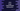# Java array copyOfRange method explanation with example## Java array copyOfRange method explanation with example:

copyOfRange method is used to copy a specific range of array to a new array. We can give the start and end index for the copy and it will copy that part of the array to a new array.

In this post, we will learn how to use copyOfRange method, its definition and example of using this method.

### Definition of copyOfRange:

copyOfRange method is defined as like below:

``public static <T> T[] copyOfRange(T[] original, int from, int to)``

Here,

• original is the original array from where we are copying the items.
• from is the start index to start the copying. It is inclusive, i.e. value at this index will be included in the final array.
• to is the end index to end the copying. It is exclusive i.e. value at this index will not be included in the final array, it will copy all just before to this index value.

#### Return value of copyOfRange:

copyOfRange creates a new array by copying the items defined by from and to and it returns the newly created array. It truncates or padded the array with zero to match the final length.

#### Exceptions:

copyOfRange can throw any of the below exceptions:

• ArrayIndexOutOfBoundsException. This exception is thrown if the value of from is invalid, e.g. if it is smaller than zero or if it is greater than the size of the original array.
• IllegalArgumentException. This exception is thrown if the value of from is greater than to.
• NullPointerException. This exception is thrown if the original array is null.

### Example of copyOfRange:

Let’s take an example of copyOfRange and see how it works:

``````import java.util.Arrays;

public class Main {
static void printArray(int[] arr) {
for (int j : arr) {
System.out.println(j);
}
}

public static void main(String[] args) {
int[] firstArr = new int[]{1, 2, 3, 4, 5};

System.out.println("Original array: ");
printArray(firstArr);

int[] copiedArr = Arrays.copyOfRange(firstArr, 0, 3);

System.out.println("New array: ");
printArray(copiedArr);
}
}``````

Here,

• printArray method is used to print an array. We are calling this method to print the arrays.
• Inside main, we have created an integer array firstArr.
• copiedArr is the new array created by using copyOfRange on firstArr. The from value is 0 and to value is 3. So, it picked the elements from index 0 to 2 from firstArr to create the copiedArr.

If you run this program, it will print the below output:

``````Original array:
1
2
3
4
5
New array:
1
2
3``````

### Example with equal from and to values:

If you pass same or equal from and to values, copyOfRange will return an empty array. For example,

``````import java.util.Arrays;

public class Main {
static void printArray(int[] arr) {
for (int j : arr) {
System.out.println(j);
}
}

public static void main(String[] args) {
int[] firstArr = new int[]{1, 2, 3, 4, 5};

System.out.println("Original array: ");
printArray(firstArr);

int[] copiedArr = Arrays.copyOfRange(firstArr, 4, 4);

System.out.println("New array: ");
printArray(copiedArr);
}
}``````

copiedArr will be an empty array since both from and to are 4 in this example.

### Example of to greater than the length of the original array:

If the to value you are passing to this method is greater than the size of the original array, it will pad the extra positions with 0. For example,

``````import java.util.Arrays;

public class Main {
static void printArray(int[] arr) {
for (int j : arr) {
System.out.println(j);
}
}

public static void main(String[] args) {
int[] firstArr = new int[]{1, 2, 3, 4, 5};

System.out.println("Original array: ");
printArray(firstArr);

int[] copiedArr = Arrays.copyOfRange(firstArr, 0, 10);

System.out.println("New array: ");
printArray(copiedArr);
}
}``````

Here, the length of the array firstArr is 5, but the value of to is 10. So, it will add zeroes to fill the extra positions.

``````Original array:
1
2
3
4
5
New array:
1
2
3
4
5
0
0
0
0
0``````

### Exception:

Let’s try with invalid from and to values to get an exception:

``int[] copiedArr = Arrays.copyOfRange(firstArr, 20, 10);``

It will throw an IllegalArgumentException.